Calculator Library
Free Online Calculators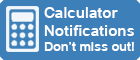Home » 80+ Calculators » Blog » How To Reset A Graphing Calculator?

Huge Domain Name Sale | Free Domains
Domain names on sale at crazy prices. Register a new domain for FREE when you buy another service.

## HOW TO RESET A GRAPHING CALCULATOR?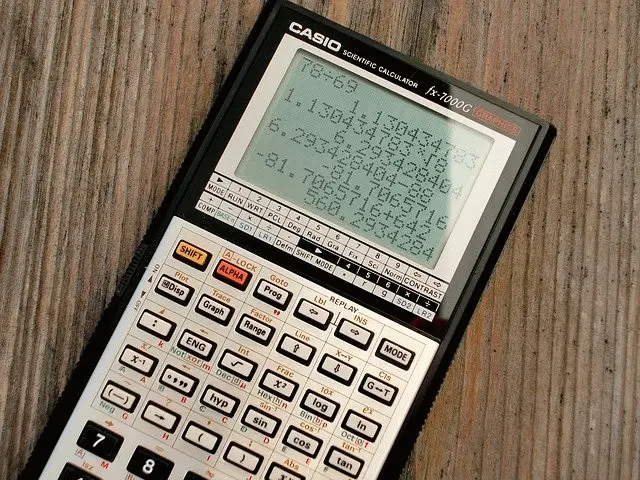If you're experiencing problems with your graphing calculator, don't worry – there is a way to reset it. In this article, we will walk you through the process of how to reset your graphing calculator. We will also provide some troubleshooting tips if you are having trouble resetting your calculator. Keep reading for more information!

## What Is A Graphing Calculator?

A graphing calculator is a type of calculator that can plot graphs and solve complex mathematical problems. Graphing calculators are usually used in math and science classes, as they can help students visualize concepts.

Many graphing calculators also have features that allow users to perform calculations, such as finding the roots of equations. The graphing calculator was originally launched in the 1980s.

The LCD screen is the most distinguishing feature of a graphing calculator. It enables the calculator to produce graphs, but it also serves as a display for all other functions. Because she can see all of her entries on it, it allows users to detect and repair mistakes better than previous calculators.

## Functions Of A Graphing Calculator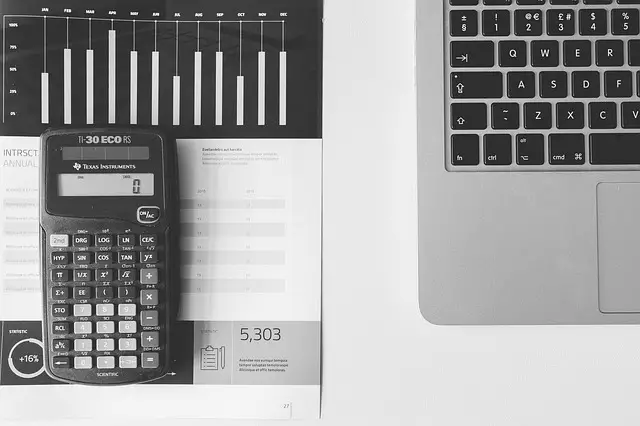Most graphing calculators can perform the following functions:

### Plot Graphs Of Equations

A graphing calculator can plot the graph of any equation. This is a useful tool for visualizing concepts in math and science. Plotting the graph of an equation can also help you solve problems. These calculators usually have an equation entry mode, where you can enter the equation that you want to graph.

### Solve Equations

A graphing calculator can also be used to solve equations. This is a useful tool for solving problems that cannot be solved by hand. To solve an equation, you will need to enter the equation into the calculator in either the equation entry mode or the graphing mode. Once the equation is entered, you can then use the calculator to find the roots of the equation.

### Geometry And Calculus

A graphing calculator can also be used for geometry and calculus. This is a useful tool for visualizing concepts in math and science. These calculators usually have a geometry mode, where you can enter the equations that you want to graph. You can also use the calculus mode to find the derivatives of functions.

### Trigonometry

A graphing calculator can also be used for trigonometry. This is a useful tool for visualizing concepts in math and science. These calculators usually have a trigonometry mode, where you can enter the equations that you want to graph. You can also use the calculator to find the roots of trigonometric functions.

### Finance & Statistics

A graphing calculator can also be used for finance. This is a useful tool for financial problems. These calculators usually have a finance mode, where you can enter the equations that you want to solve. You can also use the calculator to find the roots of financial functions.

A graphing calculator can also be used for statistics. This is a useful tool for statistical problems. These calculators usually have a statistics mode, where you can enter the equations that you want to solve. You can also use the calculator to find the roots of statistical functions.

## How To Reset Graphing Calculator?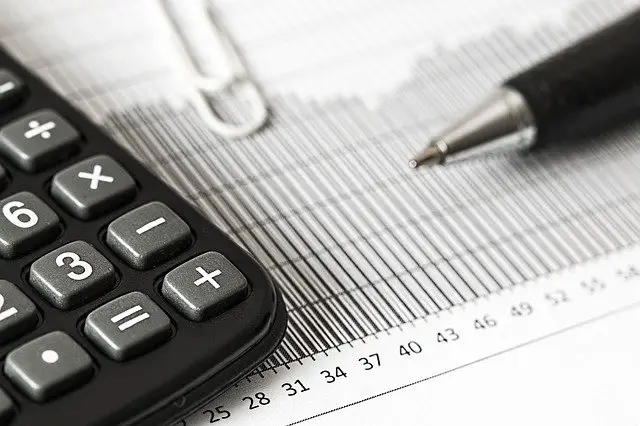If you want to how reset graphing calculator, there are a few different ways that you can do this. The most common way is to use the reset button. This button is usually located on the back of the calculator. Another way to reset graphing calculator is to remove the batteries and then replace them. This will reset the calculator to its default settings. You can also use a software program to reset the graphing calculator. This is a more advanced method and should only be used if you are familiar with how to use software programs. But this will remove all of the data stored in your archive memory and ram memory.

Now that you know how to reset graphing calculator, you can use it to solve problems. To solve a problem using graphing calculator, you will need to enter the equation that you want to graph. To do this, you will need to press the mode button. This button is usually located on the side of the calculator. You can then use the arrow keys to select the desired function. To graph an equation, you will need to press the graph button. This button is usually located on the front of the calculator.

If you want to learn more about how to use a graphing calculator, you can check out the user manual. This is a great resource for learning how to use the different features of your calculator. You can also find tutorial videos online. These videos can help you learn how to use the different features of your graphing calculator. With a little practice, you will be able to use your graphing calculator to solve problems.

## Most Popular Graphing Calculators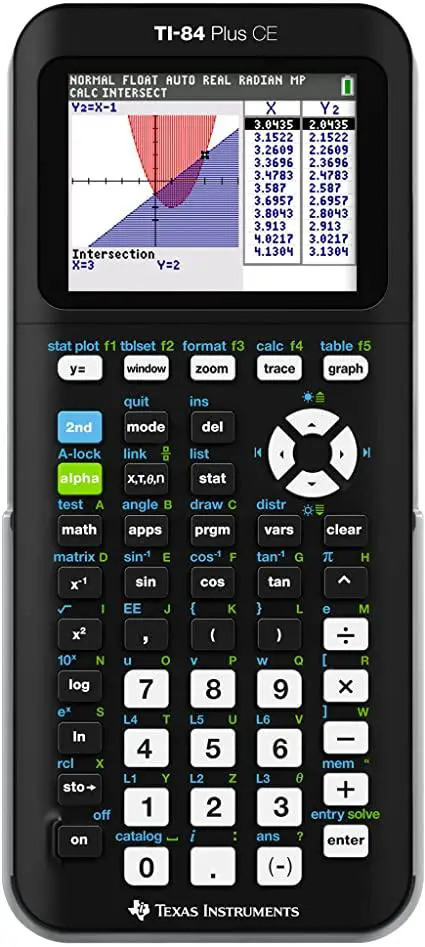There are a few different types of graphing calculators on the market. The most popular type is the TI-84 Plus graphing calculator. This calculator from Texas instruments is a great choice for solving problems in math and science. The TI-84 Plus has a color screen, which makes it easy to see the different colors of the graph. It also has a USB port, so you can connect it to your computer.

Another popular graphing calculator is the TI-Nspire. This calculator has a touchpad, which makes it easy to use. The TI-Nspire is a great choice for solving problems in math and science.

The Casio fx-9750GII is another popular graphing calculator. It is one of the most user-friendly graphing calculators on the market. It's great for beginners and has a high degree of usability. Computing speeds are quick. For a graphing calculator, it comes at a great price.

## Conclusion

If you want to how reset graphing calculator, there are a few different ways that you can do this. The most common way is to use the reset button. This button is usually located on the back of the calculator. In this article, we have also discussed a few other ways to reset the graphing calculator.

Thank you for reading this article. We hope that you have found it to be helpful.

 Previous Post« How To Put A Fraction On An Online Calculator? Next PostHow To Turn Off Sound On A Calculator? »

Copyright © Calculator Library, 1998 - 2022.
Made with ♥ in Australia.